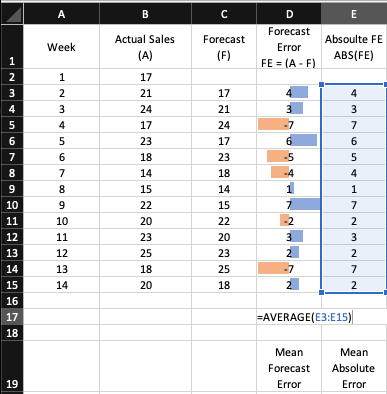top of page
Search

# Basics of Forecast AccuracyIt is near impossible to calculate the accurate forecast despite the availability of multiple forecasting methods. And it is recommended that we should run various forecast models and compare them to find out which model is giving us a more accurate forecast.

## Why do we need forecast accuracy?

For measuring accuracy, we compare the existing data with the data obtained by running the prediction model for existing periods. The difference between the actual and predicted value is also known as forecast error. Lesser the forecast error, the more accurate our model is.

By selecting the method that is most accurate for the data already known, it increases the probability of accurate future values.

## Measures of forecast accuracy

There are several measures to measure forecast accuracy:

• Mean Forecast Error (MFE)

• Mean Absolute Error (MAE) or Mean Absolute Deviation (MAD)

• Root Mean Square Error (RMSE)

• Mean Absolute Percentage Error (MAPE)

Let us consider the following table for this example.The table shows the weekly sales volume of a store. The store manager is not savvy with forecasting models, so he considered the current week’s sales as a forecast for next week. This methodology is also known as the naïve forecasting method due to the nature of simplicity.## Calculating Forecast Error

The difference between the actual value and the forecasted value is known as forecast error. So, in this example, forecast error for week 2 is

Forecast Error (week 2) = 21 – 17 = 4

A positive value of forecast error signifies that the model has underestimated the actual value of the period. A negative value of forecast error signifies that the model has overestimated the actual value of the period.

The following table calculates the forecast error for the rest of the weeks:## Mean Forecast Error

A simple measure of forecast accuracy is the mean or average of the forecast error, also known as Mean Forecast Error.

In this example, calculate the average of all the forecast errors to get mean forecast error:The MFE for this forecasting method is 0.2.

Since the MFE is positive, it signifies that the model is under-forecasting; the actual value tends to more than the forecast values. Because positive and negative forecast errors tend to offset one another, the average forecast error is likely to be small. Hence, MFE is not a particularly useful measure of forecast accuracy.

## Mean Absolute Deviation (MAD) or Mean Absolute Error (MAE)

This method avoids the problem of positive and negative forecast errors. As the name suggests, the mean absolute error is the average of the absolute values of the forecast errors.MAD for this forecast model is 4.08## Mean Squared Error (MSE)

Mean Squared Error also avoids the challenge of positive and negative forecast errors offsetting each other. It is obtained by:

• First, calculating the square of the forecast error

• Then, taking the average of the squared forecast error## Root Mean Squared Error (RMSE)

Root Mean Squared Error is the square root of Mean Squared Error (MSE). It is a useful metric for calculating forecast accuracy.RMSE for this forecast model is 4.57. It means, on average, the forecast values were 4.57 values away from the actual.

## Mean Absolute Percentage Error (MAPE)

The size of MAE or RMSE depends upon the scale of the data. As a result, it is difficult to make comparisons for a different time interval (such as comparing a method of forecasting monthly sales to a method forecasting a weekly sales volume). In such cases, we use the mean absolute percentage error (MAPE).

Steps for calculating MAPE:

• by dividing the absolute forecast error by the actual value

• calculating the average of individual absolute percentage errorThe MAPE for this model is 21%.It signifies that the 21% average deviation of the forecast from the actual value in the given model.

## How to use them?

These measures of forecast accuracy represent how well the forecasting method can predict the historical values of the time series.

Lower the values of these measures, the more accurate prediction model is.

So far, we have used the naïve forecast method for prediction. In the below examples, we have used a 3-period moving average and simple exponential smoothing for forecast, and then we compare the accuracy:

3-period moving average:Simple Exponential Smoothing (with smoothing factor = 0.20)## Comparing different forecasting models

Following table summarizes all forecast accuracy measures for all the three modes:We can conclude that forecast obtained through simple exponential smoothing has lower values for forecast accuracy measures. Hence, for predicting future values, we should pick this model among the three.

## Things to consider

Measures of forecast accuracy are critical factors in comparing different forecasting methods. Still, we must be careful not to rely too heavily upon them. Sound judgment and business knowledge that might impact the forecast should also be taken into consideration. Historical forecast accuracy is not the sole consideration, especially if the pattern exhibited by the time series is likely to change in the future.

@imVivRan

bottom of page